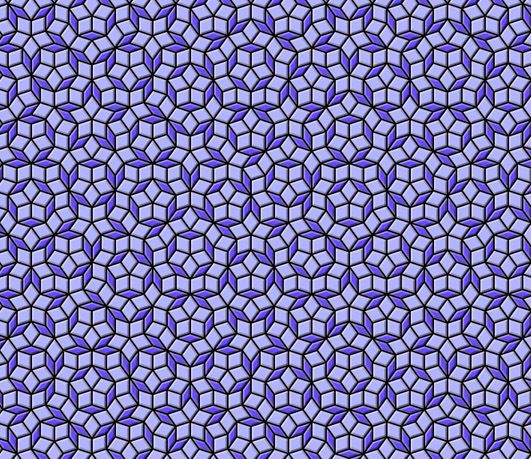### Python编程语言

Python 是一种面向对象、解释型计算机程序设计语言，由Guido van Rossum于1989年底发明，第一个公开发行版发行于1991年。Python语法简洁而清晰，具有丰富和强大的类库。它常被昵称为胶水语言，它能够把用其他语言制作的各种模块（尤其是C/C++）很轻松地联结在一起。

```_                 =
"""if!
1:"e,V=100
0,(0j-1)**-.2;
v,S=.5/ V.real,
[(0,0,4   *e,4*e*
V)];w=1     -v"def!
E(T,A,       B,C):P
,Q,R=B*w+        A*v,B*w+C
*v,A*w+B*v;retur       n[(1,Q,C,A),(1,P
,Q,B),(0,Q,P,A)]*T+[(0,C      ,R,B),(1,R,C,A)]*(1-T)"f
or!i!in!_[:11]:S    =sum([E     (*x)for    !x!in!S],[])"imp
ort!cair        o!as!O;   s=O.Ima        geSurfac
e(1,e,e)        ;c=O.Con text(s);        M,L,G=c.
move_to        ,c.line_to,c.s        et_sour
ce_rgb        a"def!z(f,a)        :f(-a.
imag,a.    real-e-e)"for!T,A,B,C!in[i    !for!i!
in!S!if!i[""";exec(reduce(lambda x,i:x.replace(chr
(i),"n "[34-i:]),  range(  35),_+"""0]]:z(M,A
);z(L,B);z     (L,C);     c.close_pa
th()"G       (.4,.3       ,1);c.
paint(       );G(.7       ,.7,1)
;c.fil       l()"fo       r!i!in
!range       (9):"!       g=1-i/
8;d=i/     4*g;G(d,d,d,     1-g*.8
)"!def   !y(f,a):z(f,a+(1+2j)*(   1j**(i
/2.))*g)"!for!T,A,B,C!in!S:y(M,C);y(L,A);y(M
,A);y(L,B)"!c.st      roke()"s.write_t
o_png('pen            rose.png')
"""                    ))
```python转换字符串为摩尔斯电码的方法